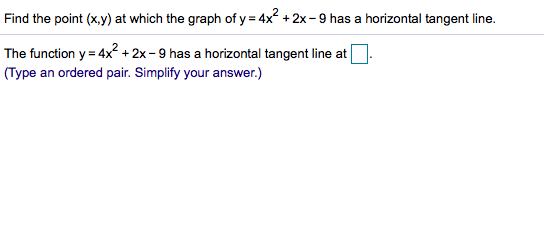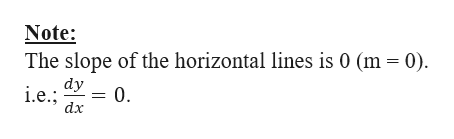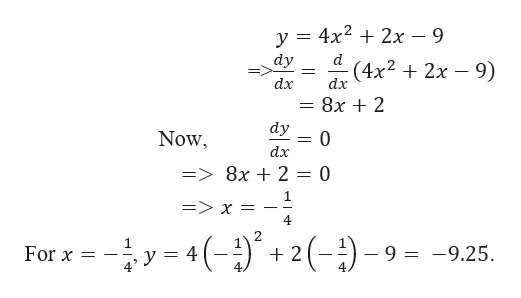# Find the point (x,y) at which the graph of y 4x2x - 9 has a horizontal tangent line.=The function y 4x2 +2x -9 has a horizontal tangent line at(Type an ordered pair. Simplify your answer.)

Question
1 viewshelp_outlineImage TranscriptioncloseFind the point (x,y) at which the graph of y 4x 2x - 9 has a horizontal tangent line. = The function y 4x2 +2x -9 has a horizontal tangent line at (Type an ordered pair. Simplify your answer.) fullscreen
check_circle

Step 1

We need to find the point (x, y) at which the graph of y = 4x2+2x-9 has a horizontal tangent line.help_outlineImage TranscriptioncloseNote: The slope of the horizontal lines is 0 (m 0) dy = 0 i.e. dx fullscreen
Step 2

Here,

...help_outlineImage Transcriptionclosey 4x22x - 9 dy d (4x22x 9) dx dx 8x 2 dy 0 Now dx => 8x + 2 = 0 1 => x 4 1 - 9 = -9.25 For x = 4 2 5- () fullscreen

### Want to see the full answer?

See Solution

#### Want to see this answer and more?

Solutions are written by subject experts who are available 24/7. Questions are typically answered within 1 hour.*

See Solution
*Response times may vary by subject and question.
Tagged in

### Derivative